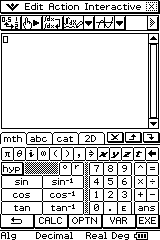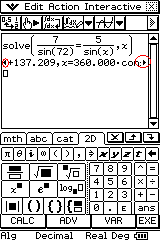## Thursday, January 22, 2009

### Trigonometry and CAS calculator I

During exams last year we noticed that those who had CAS calculators were not using them for Trigonometry problems. During summer school we sought to rectify this. To start with we looked at properties of triangles.

Students stated that the calculator would give no solution when solutions existed. This sounded doubtful but I had a good idea where they were going wrong.

I started with the following. Take three lines, 4cm, 2cm, 1cm. Now obviously this can't make a triangle. Right?No matter how we change the angles at A & B they cannot form a triangle. This was to inform students that a no solution result in their calculator had meaning.

So now we had a look at a problem that they were having difficulty with.In their work pads they had written A=x, a=5cm, B=72°, b=72 and labelled the triangle correctly. So far so good.

They could tell it was a sine rule problem but had difficulty entering it into the calculator. Where did the sin, cos, tan buttons go from the calculator?

The first thing to do is find the sine function. Open the soft keyboard, select the mth tab and press the Trig button at the bottom of the keyboard.Next enter the sine equation with the substituted values. They needed the fraction template inside the soft keyboard under 2DHitting execute at this point gives no solution. Huh?

Well.. we still have to solve for x. So highlight the equation and go to the interactive menu (in the menu bar at the top of the work pane), select Advanced and then solve. The Equation should be there and the variable listed should be x.After a rather long wait a huge expression appeared with a strange looking answer.

I'll rekey it here as the whole answer does not appear on the work pane on the calculator.

{x=360.00.constn(1)+137.209, x=360.00.constn(2)+42.791}

The answers are the two bits in red (at this point we had a bit of a chat about the ambiguous case with the sine rule). To get to the answer you have to navigate with the style and the left/right arrows at the edges of the equation.So the answer is x=137° or x=43° (0 d.p.)

We then drew these triangles to give students a better understanding of the ambiguous case.

In the next example we'll look at the perils of rounding and go back to the case above with the impossible triangle.

Here is a link to other CAS calculator posts.